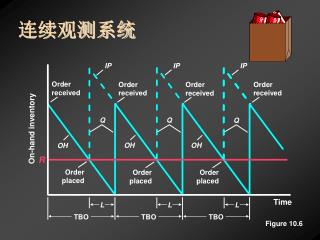# 连续观测系统 - PowerPoint PPT PresentationDownload PresentationDownload Presentation## 连续观测系统

- - - - - - - - - - - - - - - - - - - - - - - - - - - E N D - - - - - - - - - - - - - - - - - - - - - - - - - - -
##### Presentation Transcript

1. Soup Soup Soup IP IP IP Order received Order received Order received Order received Q Q Q On-hand inventory OH OH OH R Order placed Order placed Order placed Time L L L TBO TBO TBO Figure 10.6 连续观测系统

2. IP IP Order received Order received Order received Order received Q Q Q OH On-hand inventory R Order placed Order placed Order placed Time L1 L2 L3 TBO1 TBO2 TBO3 不确定需求

3. 再订货点/ 安全库存 Average demand during lead time

4. Cycle-service level = 85% Probability of stockout (1.0 – 0.85 = 0.15) Average demand during lead time R zL 再订货点/ 安全库存

5. Cycle-service level = 85% Probability of stockout (1.0 – 0.85 = 0.15) Average demand during lead time R zL Safety Stock/R Safety stock = zsL = 2.33(22) = 51.3 = 51 boxes Reorder point = ADDLT + SS = 250 + 51 = 301 boxes 再订货点/ 安全库存

6. st = 15 st = 15 st = 15 st = 26 + 75 Demand for week 1 + 225 Demand for three-week lead time 75 Demand for week 2 = 75 Demand for week 3 提前期分布

7. T On-hand inventory Time P P 定期观测系统

8. T Q1 On-hand inventory Order placed Time P P 定期观测系统

9. T Q1 On-hand inventory Order placed L Time P P 定期观测系统

10. T Order received Q1 On-hand inventory Order placed L Time P P 定期观测系统

11. T Order received Order received Order received Q3 Q1 Q2 On-hand inventory Order placed Order placed L L L Time P P 定期观测系统

12. T IP IP IP Order received Order received Order received Q3 Q1 OH OH Q2 IP1 IP3 IP2 On-hand inventory Order placed Order placed L L L Time P P Protection interval 定期观测系统

13. T IP IP IP Order received Order received Order received Bird feeder—Calculating P and T Q3 Q1 OH OH Q2 IP1 IP3 IP2 On-hand inventory EOQ D 75 936 P = (52) = (52) = 4.2 or 4 weeks Order placed Order placed P+L = st P + L = 5 6 = 12 units L L L Time P P Protection interval 定期观测系统 t= 5 units L = 2 weeks cycle/service level = 90% EOQ = 75 units D = (18 units/week)(52 weeks) = 936 units T = Average demand during the protection interval + Safety stock = d (P + L) + zsP + L = (18 units/week)(6 weeks) + 1.28(12 units) = 123 units

14. T IP IP IP Order received Order received Order received Bird feeder—Calculating P and T Q3 Q1 OH OH Q2 IP1 IP3 IP2 On-hand inventory 4(18) 2 936 4(18) C = (\$15) + (\$45) + 15(\$15) Order placed Order placed L L L Time P P Protection interval 定期观测系统 t= 5 units L = 2 weeks cycle/service level = 90% EOQ = 75 units D = (18 units/week)(52 weeks) = 936 units P = 4 weeks T = 123 units C = \$540 + \$585 + \$225 = \$1350

15. P 系统 • 库存量补充定期进行，系统管理简单 • 从同一供应商订购多种物品，可以把订单合为一份采购单 • 只有在进行观测时，才需要了解库存状况 • 每种物品的观测频数可能各不相同，可减少总的订购及库存持有成本 • 订货批量固定，如果批量足够大，可能获得数量折扣 • 较低的安全库存量取得了节约的效果。 Q系统和P系统比较 Q 系统